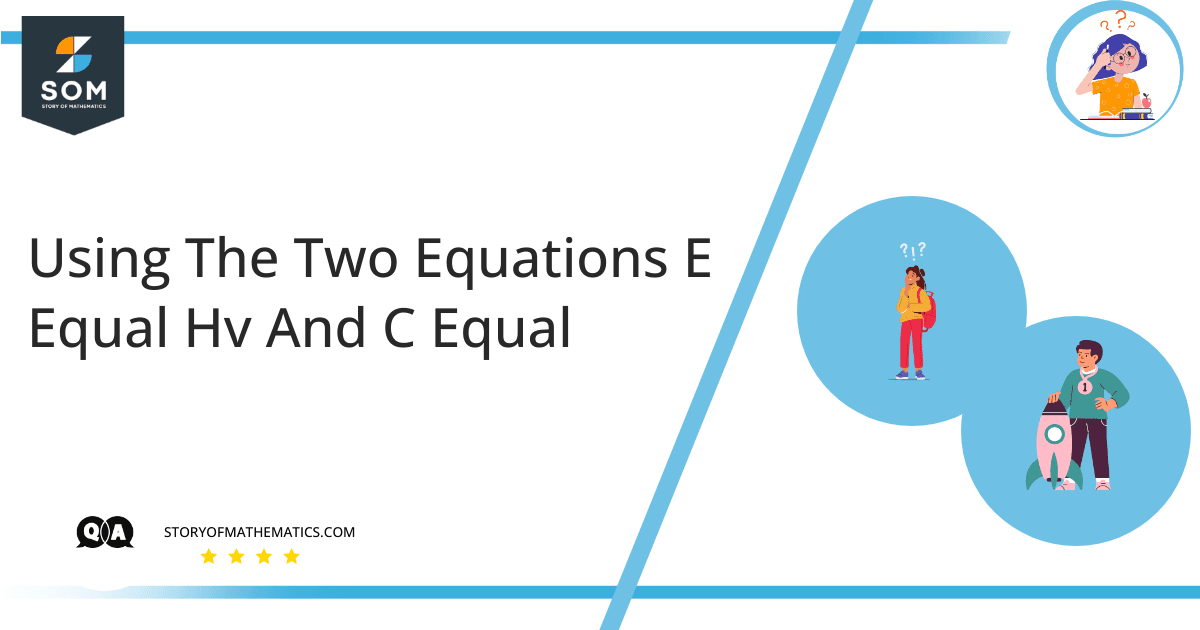# Using the two equations E=hv and c=lambda v derive an equation expressing E in terms of h,c and lambda.This question aims to express the quantum of energy $(E)$ in terms of the speed of light $(c)$, the wavelength $(\lambda)$, and Planck’s constant $(h)$.

The frequency can be expressed as the number of oscillations in one unit of time and it is calculated in Hz (hertz). The wavelength is regarded as the measure of length between two points in sequence. As a result, two adjoining troughs and peaks on a wave are isolated by one complete wavelength. The Greek letter $\lambda$ is commonly utilized to represent the wavelength of a wave.

For instance, the speed of traveling waves and wavelength is proportional to the frequency. When a wave moves rapidly, the number of complete wave phases completed in one second is greater than when the wave moves slower. As a result, the speed at which a wave moves is a critical factor in working out its frequency. In physics and chemistry, quantum signifies a specific package of energy or matter. It is the smallest quantity of energy required for a progression or the smallest value of any substantial resource in interaction as utilized in operation.

Let $\lambda$ be the wavelength, $c$ be the speed of light, and $v$ be the frequency. The frequency and wavelength are then related as:

$c=\lambda v$                                          (1)

Also, if $E$ is the quantum of energy, and $h$ is the Planck’s constant, then the quantum of energy and frequency of radiation are related as:

$E=hv$                                                    (2)

Now from (1):

$v=\dfrac{c}{\lambda}$

Substitute this in equation (2) to get:

$E=h\left(\dfrac{c}{\lambda}\right)$

$E=\dfrac{hc}{\lambda}$

## Example 1

A ray of light has the wavelength $400\,nm$, find its frequency.

### Solution

Since  $c=\lambda v$

Therefore,  $v=\dfrac{c}{\lambda}$

It is well known that the speed of light is $3\times 10^8\,m/s$. So using the given values in the above formula, we get:

$v=\dfrac{3\times 10^8\,m/s}{400\times 10^{-9}\,m}$

$v=0.0075\times 10^{17}\,Hz$

$v=7.5\times 10^{14}\,Hz$

## Example 2

A ray of light has the frequency $1.5\times 10^{2}\, Hz$, find its wavelength.

### Solution

Since  $c=\lambda v$

Therefore, $\lambda=\dfrac{c}{v}$

It is well known that the speed of light is $3\times 10^8\,m/s$. So using the given values in the above formula, we get:

$\lambda=\dfrac{3\times 10^8\,m/s}{1.5\times 10^{2}\,Hz}$

$\lambda= 2\times 10^{6}\,m$

## Example 3

The Planck’s constant is assumed to be $6.626\times 10^{-34}\,J\,s$.  Calculate $E$ if the frequency is $2.3\times 10^9\,Hz$.

### Solution

Given that:

$h=6.626\times 10^{-34}\,J\,s$

$v=2.3\times 10^9\,Hz$

To find $E$.

Since we know that:

$E=hv$

Substituting the given information:

$E=(6.626\times 10^{-34}\,J\,s)(2.3\times 10^9\,Hz)$

$E=15.24\times 10^{-25}\,J$